# Electromagnetic Wave Equation

The wave equation for a plane electric wave traveling in the x direction in space is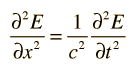with the same form applying to the magnetic field wave in a plane perpendicular the electric field. Both the electric field and the magnetic field are perpendicular to the direction of travel x. The symbol c represents the speed of light or other electromagnetic waves. The wave equation for electromagnetic waves arises from Maxwell's equations. The form of a plane wave solution for the electric field is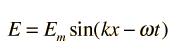and that for the magnetic fieldTo be consistent with Maxwell's equations, these solutions must be related byThe magnetic field B is perpendicular to the electric field E in the orientation where the vector product E x B is in the direction of the propagation of the wave.

 Transport of energy by electromagnetic waves
Index

Wave concepts

Electromagnetic wave concepts

Electromagnetic spectrum

 HyperPhysics*****Electricity and Magnetism R Nave
Go Back

# Energy in Electromagnetic Waves

Electromagnetic waves carry energy as they travel through empty space. There is an energy density associated with both the electric field E and the magnetic field B. The rate of energy transport per unit area is described by the vector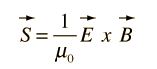which is called the Poynting vector. This expression is a vector product, and since the magnetic field is perpendicular to the electric field, the magnitude can be written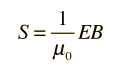The rate of energy transport S is perpendicular to both E and B and in the direction of propagation of the wave. A condition of the wave solution for a plane wave is Bm = Em/c so that the average intensity for a plane wave can be written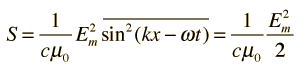This makes use of the fact that the average of the square of a sinusoidal function over a whole number of periods is just 1/2.

Index

Wave concepts

Electromagnetic wave concepts

 HyperPhysics*****Electricity and Magnetism R Nave
Go Back